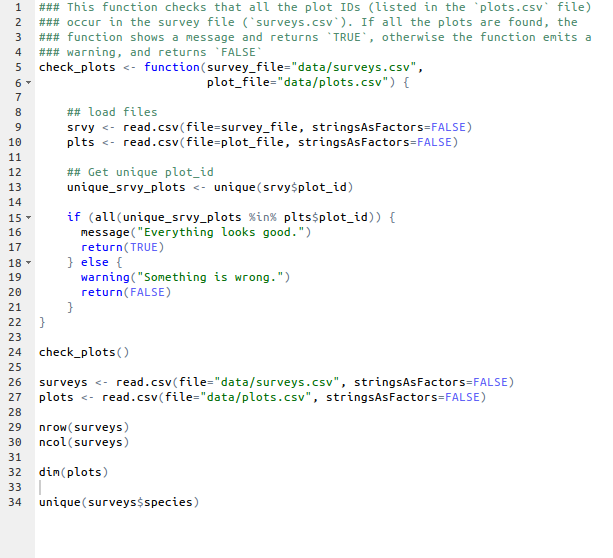## Learning Objectives

• Familiarize participants with R syntax
• Understand the concepts of objects and assignment
• Understand the concepts of vector and data types
• Get exposed to a few functions

## The R syntax

Start by showing an example of a script

• Point to the different parts:
• a function
• the assignment operator `<-`
• the `=` for arguments
• the comments `#` and how they are used to document function and its content
• the `\$` operator
• Point to indentation and consistency in spacing to improve clarity## Creating objects

You can get output from R simply by typing in math in the console

``````3 + 5
12/7
``````

However, to do useful and interesting things, we need to assign values to objects. To create objects, we need to give it a name followed by the assignment operator `<-` and the value we want to give it:

``````weight_kg <- 55
``````

Objects can be given any name such as `x`, `current_temperature`, or `subject_id`. You want your object names to be explicit and not too long. They cannot start with a number (`2x` is not valid but `x2` is). R is case sensitive (e.g., `weight_kg` is different from `Weight_kg`). There are some names that cannot be used because they represent the names of fundamental functions in R (e.g., `if`, `else`, `for`, see here for a complete list). In general, even if it's allowed, it's best to not use other function names (e.g., `c`, `T`, `mean`, `data`, `df`, `weights`). In doubt check the help to see if the name is already in use. It's also best to avoid dots (`.`) within a variable name as in `my.dataset`. There are many functions in R with dots in their names for historical reasons, but because dots have a special meaning in R (for methods) and other programming languages, it's best to avoid them. It is also recommended to use nouns for variable names, and verbs for function names. It's important to be consistent in the styling of your code (where you put spaces, how you name variable, etc.). In R, two popular style guides are Hadley Wickham's and Google's.

When assigning a value to an object, R does not print anything. You can force to print the value by using parentheses or by typing the name:

``````(weight_kg <- 55)
weight_kg
``````

Now that R has `weight_kg` in memory, we can do arithmetic with it. For instance, we may want to convert this weight in pounds (weight in pounds is 2.2 times the weight in kg):

``````2.2 * weight_kg
``````

We can also change a variable's value by assigning it a new one:

``````weight_kg <- 57.5
2.2 * weight_kg
``````

This means that assigning a value to one variable does not change the values of other variables. For example, let's store the animal's weight in pounds in a variable.

``````weight_lb <- 2.2 * weight_kg
``````

and then change `weight_kg` to 100.

``````weight_kg <- 100
``````

What do you think is the current content of the object `weight_lb`? 126.5 or 200?

### Exercise

What are the values after each statement in the following?

``````mass <- 47.5           # mass?
age  <- 122            # age?
mass <- mass * 2.0     # mass?
age  <- age - 20       # age?
massIndex <- mass/age  # massIndex?
``````

## Vectors and data types

A vector is the most common and basic data structure in R, and is pretty much the workhorse of R. It's a group of values, mainly either numbers or characters. You can assign this list of values to a variable, just like you would for one item. For example we can create a vector of animal weights:

``````weights <- c(50, 60, 65, 82)
weights
``````

A vector can also contain characters:

``````animals <- c("mouse", "rat", "dog")
animals
``````

There are many functions that allow you to inspect the content of a vector. `length()` tells you how many elements are in a particular vector:

``````length(weights)
length(animals)
``````

`class()` indicates the class (the type of element) of an object:

``````class(weights)
class(animals)
``````

The function `str()` provides an overview of the object and the elements it contains. It is a really useful function when working with large and complex objects:

``````str(weights)
str(animals)
``````

You can add elements to your vector simply by using the `c()` function:

``````weights <- c(weights, 90) # adding at the end
weights <- c(30, weights) # adding at the beginning
weights
``````

What happens here is that we take the original vector `weights`, and we are adding another item first to the end of the other ones, and then another item at the beginning. We can do this over and over again to build a vector or a dataset. As we program, this may be useful to autoupdate results that we are collecting or calculating.

We just saw 2 of the 6 data types that R uses: `"character"` and `"numeric"`. The other 4 are:

• `"logical"` for `TRUE` and `FALSE` (the boolean data type)
• `"integer"` for integer numbers (e.g., `2L`, the `L` indicates to R that it's an integer)
• `"complex"` to represent complex numbers with real and imaginary parts (e.g., `1+4i`) and that's all we're going to say about them
• `"raw"` that we won't discuss further

Vectors are one of the many data structures that R uses. Other important ones are lists (`list`), matrices (`matrix`), data frames (`data.frame`) and factors (`factor`).

Another way to keep track of data types is by their content (homogeneous, i.e. same type or different i.e. heterogeneous)

dimension Homogeneous (same data type) Heterogeneous (different data types)
1d Atomic vector List
2d Matrix Data frame
nd Array

We are now going to use our “surveys” dataset to explore the `data.frame` data structure.